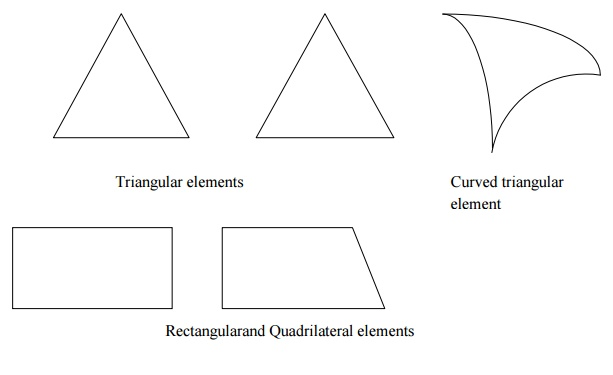Home | | Structural Analysis II | Important Questions and Answers: Structural Analysis- Finite Element Method

# Important Questions and Answers: Structural Analysis- Finite Element Method

Civil - Structural Analysis - Finite Element Method

FINITE ELEMENT METHOD

1. What is meant by Finite element method?

Finite element method (FEM)is a numerical technique for solving boundary value problems in which a large domain is divided into smaller pieces or elements. The solution is determined by asuuming certain ploynomials. The small pieces are called finite element and the polynomials are called shape functions.

2. List out the advantages of FEM.

Since the properties of each element are evaluated separately differnt material properties can be incorporated for each element.

There is no restriction in the shape of the medium.

Any type of boundary condition can be adopted.

3. List out the disadvantages of FEM.

The computational cost is high.

The solution is approximate and several checks are required.

4. Mention the various coordinates in FEM.

Local or element coordinates

Natural coodinates

Simple natural coodinates

Area coordiantesor Triangular coordiantes

Generalised coordinates

5. What are the basic steps in FEM?

Discretization of the structure

Selection of suitable displacement fuction

Finding the element properties

Assembling the element properties

Applying the boundary conditions

Solving the system of equations

6. What is meant by discretization?

Discretization is the process of subdividing the given body into a number of elements which results in a system of equivalent finite elements.

7. What are the factors governing the selection of finite elements?

The geometry of the body

The number of independent space coordinates

The nature of stress variation expected

8. Define displacement function.

Displcement function is defined as simple functions which are assumed to approximate the displacements for each element. They may assumed in the form of poynomials, or trignometrical functions.

9. Briefly explain a few terminology used in FEM.

The various terms used in FEM are explained below.

Finite element-Small elements used for subdividing the given domain tobe  analysed are called finite elements. The seelements may be 1D, 2D or 3D elements depend in on the type of structure.

Nodes and nodal points- The intersection of the differnt sides of elements are called nodes. Nodes are of two types - external nodes and internal nodes.

External nodes - The nodal point connecting adjacent elements.

Internal nodes- The extra nodes used to increase the accuracy of solution.

Nodal lines - The interface between elements are called nodal lines.

Continuum- The domain in which matter exists at every point is called a continuum. It can be assumed as having infinite number of connected particles.

Primary unknowns- The main unknowns involved in the formulation of the element properties are known as primary unknowns.

Secondary unknowns- These unknowns are derived from primary unknowns are known as secondary unknowns. In displacement formulations, displacements are treated as primary unknowns and stress, strain, moments and shear force are treated as secondary unknowns.

10.            What are differnt types of elements used in FEM?

The various elements used in FEM are classified as:

One dimensional elements(1D elements)

Two dimensional elements(2D elements)

Three dimensional elements(3D elements)

11.            Whatare1-D elements? Give examples.

Elements having a minimum of two nodes are called 1D elements. Beams are usually approximated with 1Delements. These may be straight or curved. There can be additional nodes within the element.12.            Whatare2-D elements? Give examples.

A plane wall, plate, diaphragm, slab, shell etc. can be approximated as an assemblage of 2-D elements. Most commonly used elements are triangular, rectangular and quadrilateral elements.13.            What are 3-D elements? Give examples.

3-D elements are used for modeling solid bodies and the various 3-Delements are tetrahedron, hexa hedron, and curved rectangular solid.

14.            What are axisymmetric elements?

Axisymmetric elements are obtained by rotatinga1-D line about an axis. Axisymmetric elements are shown in the figure below.

15.            Define Shape function.

Shape function is also called an approximate function or an interpolation function whose value is equal to unity at the node considered and zeros at all other nodes. Shape function is represented by Ni where i =nodeno.

16.            What are the properties of shape functions?

The properties of shape functions are:

Theno of shape functions will be equal to theno of nodes present in the element.

Shape function will have a unit value at the node considered and zero value at other nodes.

The sum of all the shape function is equal to 1. i. e. SNi =1

17.            Define aspect ratio.

Element aspect ratio is defined as the ratio of the largest dimension of the element to its smallest dimension.

18.            What are possible locations for nodes?

The possible locations for nodes are:

Point of application of concentrated load.

Location where there is a change in intensity of loads

Locations where there are discontinuities in the geometry of the structure

Interfaces between materials of different properties.

19.            What are the characteristics of displacement functions?

Displacement functions should have the following characteristics:

The displacement field should be continuous.

The displacement function should be compatible between adjacent elements

The displacement field must represent constant strain states of elements

The displacement function must represent rigid body displacements of an element.

20.            What is meant by plane strain condition?

Plane strain is a state of strain in which normal strain and shear strain directed perpendicular to the plane of body is assumed to be zero.

Study Material, Lecturing Notes, Assignment, Reference, Wiki description explanation, brief detail
Civil : Structural Analysis : Finite Element Method : Important Questions and Answers: Structural Analysis- Finite Element Method |

Related Topics